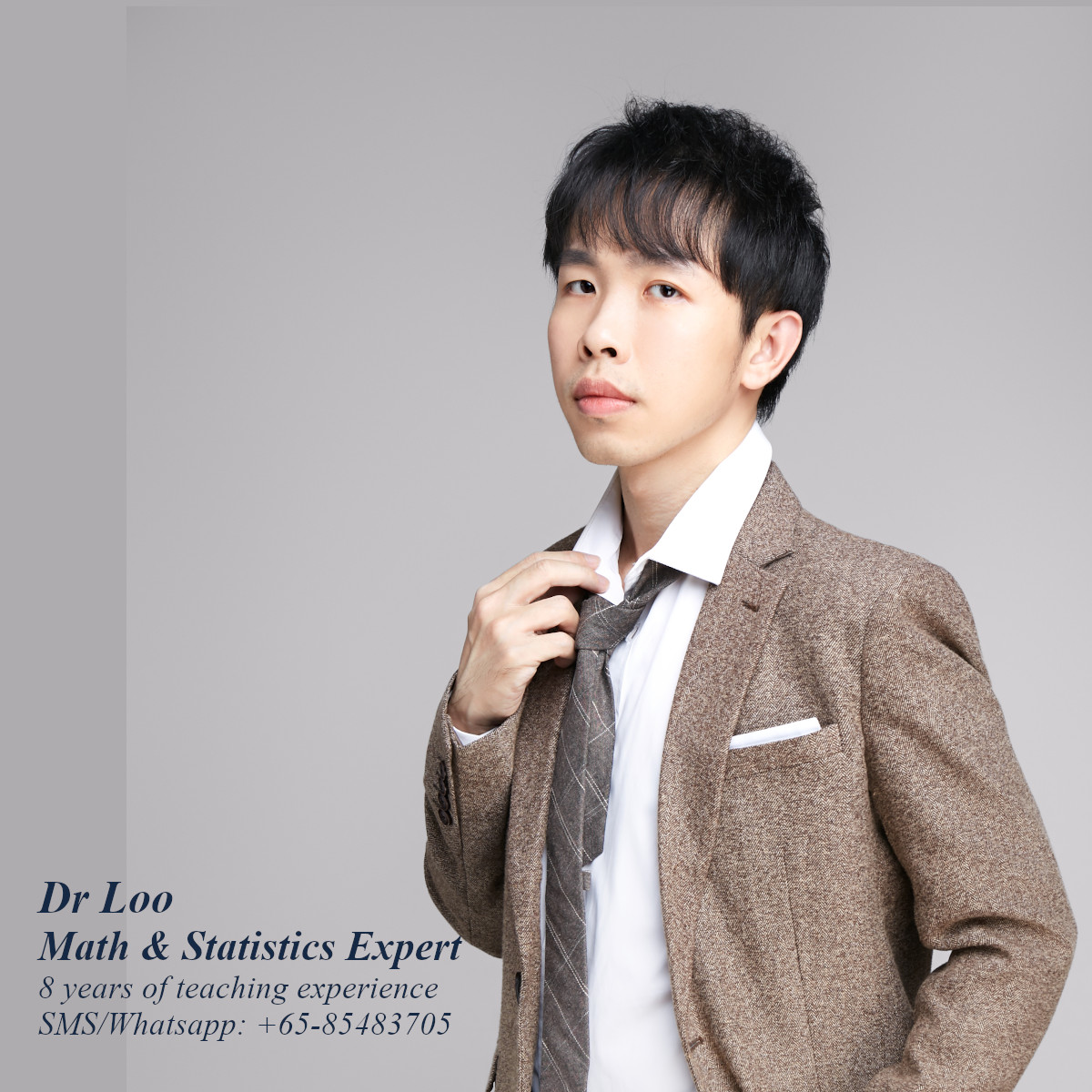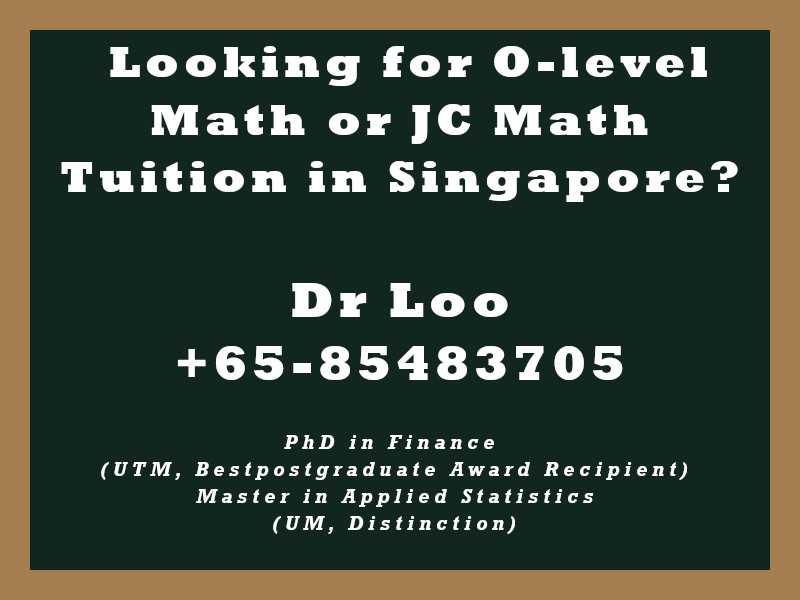+65-85483705

# Correlation Test

### Purpose of the test

To measure the relationship between two variables. Students will normally learn the formula in calculating correlation in A-level mathematics class. However, the basic concept on the correlation which is the scatter plot maybe be introduce during the O-level mathematics lessons.

## Formula

Correlation = Using the covariance of the two variables, x & y and divide it by the standard deviation of x and standard deviation of y.

## To determine the siginificance of the correlation value

Taking the sample size, n, then minus it with 2 in order to obtain the degree of freedom in order to obtain the critical value from the statistics table. df = n – 2.

Question

Calcuate the correlation values for the following set of the variables.

1. The correlation between Variable 5 & Variable 6.

 variable 5 variable 6 1 1 5 5 3 3 3 4 2 3 5 1 3 2 2 1 2 5 5 3 4 4 4 4 4 5 4 5

2. The correlation between Variable 7 & Variable 8.

 variable 7 variable 8 5 2 5 4 3 0 1 5 0 2 3 5 2 3 5 2 2 5 1 2 5 1 2 5 0 5 2 1

3. The correlation between Variable 1 & Variable 2.

 variable 1 variable 2 1 4 1 4 4 2 3 4 5 1 4 2 1 2 0 0 1 1 5 3 4 2 4 4 5 5 0 4

4. The correlation between Variable 3 & Variable 4.

 variable 3 variable 4 4 4 5 2 1 1 1 2 5 0 0 0 5 2 1 3 5 0 1 2 5 4 5 3 3 4 2 2

5. The correlation between variable 9 & variable 10.

 variable 9 variable 10 5 1 1 5 1 1 4 4 3 4 0 0 3 2 4 2 5 1 0 2 3 4 4 3 2 3 4 5

• Correlation between variable 1 & variable 2 = 0.129
• Correlation between variable 3 & variable 4 = 0.16
• Correlation between variable 5 & variable 6 = 0.335
• Correlation between variable 7 & variable 8 = -0.304
• Correlation between variable 9 & variable 10 = 0.138

## About the Singapore Math Tutor - Dr Loo

I am a PhD holder with 9 years of teaching experience on teaching quantitative subjects at secondary school and university levels. My expertise are mathematics, statistics, econometrics, finance and machine learning.

Currently I do provide consultation and tuition for math tuition in Singapore. If you are looking for secondary math tutor in Singapore, JC H2 math tutor or statistics tutor in Singapore, please feel free to contact me at +65-85483705 (SMS/Whatsapp).

Among the Singapore math tuition that I provide, this includes Singapore secondary math tuition (E-math tuition & A-math tuition) and also JC Math Tuition (H1 Math Tuition & H2 Math Tuition). On the other hand, my statistics tuition in Singapore mostly focus on pre-university level until postgraduate level. For those who are looking for online tutoring service, the online O-level math tuition and online A-level math tuition are also available.## For those who are looking for math tution in Singapore

Need help with this topic? I do provide mathematics home tuition in Singapore for O-level math and also JC H2 math. In addition, online math tutoring is available as well. Feel free to contact me if you would like to know further.